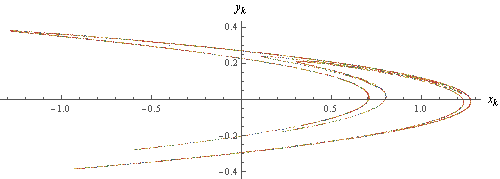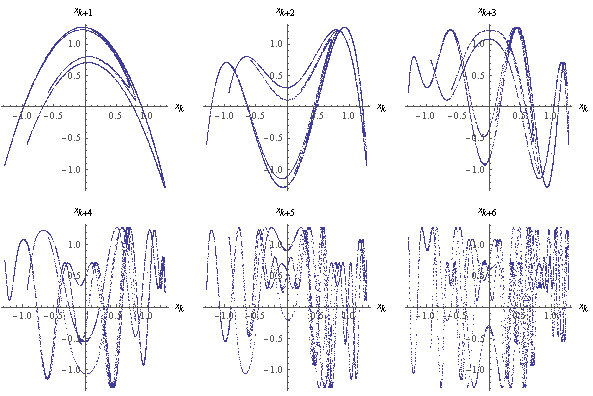# Hénon Attractor

The Hénon attractor is the orbit of the iteration H(x, y) = (y + 1 − α x2, β x).

Here are the first 5,000 iterates of H, with α = 1.4 and β = 0.3.Correlations between x-values with lags one through six:Designed and rendered using Mathematica 3.0 for the Apple Macintosh, based on images from Peitgen et al, Chaos and Fractals, Springer-Verlag, 1992.

© 1997–2019 by Robert Dickau

[ home ] || [ 97???? ]

www.robertdickau.com/henon.html